• Call Now

1800-102-2727•

# Graphs: Position-Time, Velocity-Time, Inter-Conversion, Practice Problems and FAQs

You ride to school by bike everyday. You start from rest, pedal faster, reach a certain velocity say 5 ms-1, in 10 s. Would you be able to find the displacement in 10 s? Short answer : yes, if you applied equations of motion. Another approach would be to take a pen and paper,  plot a simple graph with velocity on one axis and time on the other; the area under such a  graph would give displacement. On the other hand, plotting graphs of displacement x and time t  gives us an idea about the velocity.

A graph plotted between velocity V on the y - axis and time t on the  x - axis  is called velocity time graph or  v - t graph. Similarly, a graph plotted between position x on the vertical axis and time x on the horizontal axis is called position- time graph. With the information of v - t graph, both displacement and acceleration can be calculated. Before we dive into the details, one must understand two vital terms: slope and area. Slope is defined as the tangent of the angle which the line or tangent to the curve makes with the +Ve direction of x - axis; in the following graph for instance; slope of the curve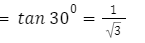slope of x-t curve gives velocity

Area under the graph is the area the curve encloses with x - axis. Area under the v - t graph gives the displacement of the bodyArea under the v-t curve gives displacement

## Displacement Time Graph

•  Graph plotted between position/displacement on the y - axis and time t on the x - axis is called position/displacement - time or x - t graph.
• Slope of the x - t   graph gives velocity.

The x - t graph for various cases is shown.Note: If acceleration is a function of position or time, then such acceleration is said to be non uniform.

## Velocity Time Graph

• A graph plotted between velocity v on the y - axis and time t on the  x - axis is called the v - t graph.
• Slope of the v - t graph gives acceleration
• Area under the v - t graph gives displacement.

The v - t graph for different cases is as shown.Note:

• The mathematical interpretation of slope of a graph is its derivative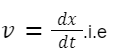.i.e differentiating displacement x wrt time t gives velocity. The same applies for acceleration;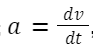, differentiating velocity v wrt time t gives acceleration a.
•  Integration refers to finding area under the graph. For example;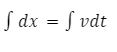i.e area under v - t graph gives the displacement.

## Practice Problems of Graphs

Question 1. A person walks 10 m in the positive direction in 5 seconds, then stops for 2  seconds, and then walks 15 m in the negative direction for 5 seconds. Draw his displacement-time graph.

Answer:  The motion can be summarized as follows:

(i) 10 m in the positive direction from 0 to 5 seconds.

(ii) At rest from t = 5 to t = 7 s

(iii)15 m in the negative direction from t = 7 to t = 12 s

The following diagram represents his motionQuestion 2. The x - t (position time graph) of an object moving in a straight line is shown. Calculate the average velocity in the time interval

(i)t = 2 to t = 4 s

(ii)t = 4s to t = 6 sAnswer: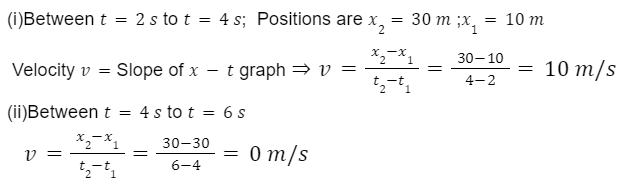Question 3. Given below is a  v - tgraph, find the (i) displacement in first 3  seconds (ii) acceleration in first 3 seconds.Answer: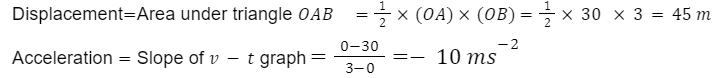Question 4. From the displacement time graph given below, find velocity?## FAQs of Graphs

Question 1. Draw the v - t graph for a stone thrown upwards;

Answer: Acceleration a = - g for a stone thrown up;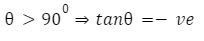Question 2. Draw the v - t graph for a ball thrown downwards from the top of a tower.

Answer: For a ball thrown downwards;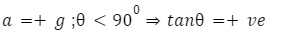Question 3. How to find acceleration from v - t graph?
Slope of the v - t graph gives acceleration a.

Question 4.  How to find displacement from v - t graph?
Area under the v - t graph gives displacement.

## NCERT Class 11 Physics Chapters

 Physical World Units and Measurements Motion in a Straight Line Motion in a Plane Laws of Motion Work Energy and Power Particles and Rotational Motion Gravitation Mechanical Properties of Solids Mechanical Properties in Liquids Thermal Properties of Matter Thermodynamics Kinetic Theory Oscillations WavesTalk to our expert
Resend OTP Timer =
By submitting up, I agree to receive all the Whatsapp communication on my registered number and Aakash terms and conditions and privacy policy